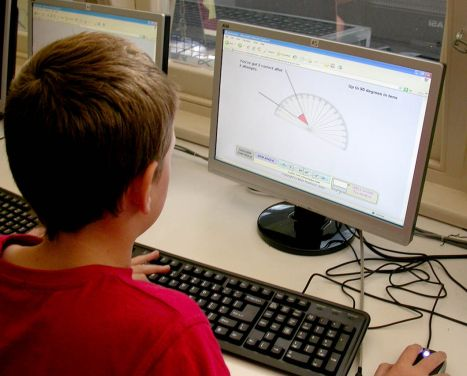# Why do computers suck at MathComputers are built using maths. Every program that is written for them generally uses a lot of math and math is the language that we use to communicate with computers. It thus becomes very perplexing that computer programs like calculator and Excel and powerful search engines too suck at maths. In fact, calculators have been known to screw up basic computations like 12.52 MINUS 12.51 (Windows 3.11 calculator actually returns the result as 0.00 instead of 0.01). We try to explain why computers suck at maths.

Numbers are harder to represent on computers than most people believe

For a system that uses numbers (0 and 1 to be precise) for every bit of its operation, it is indeed interesting to note that computers find it harder to represent numbers than they do letters of the alphabet. The errors caused by seemingly minor computational challenges appear from what is called a floating point number. A standard one of these requires precision to around 16 decimal places! We’re not even going to try and explain what kinds of calculations would need such precision but we will tell you that it poses a problem significant enough to throw off basic mathematical calculations. This basically means that computers are so smart that they get the basics wrong far too often!

0.999 infinitely repeating equals 1

A widely accepted mathematical theory states that when the figure 0.999 repeats itself infinitely, it actually denotes a real number that equals 1. Computers know this theory and employ it far too often for their own good. And that is one concept that leads computers to screw up its level of precision ever so often.

Floating-Point Arithmetic and number approximation issues

Like we told you before, floating point numbers are precise to 16 decimal places and even mathematicians give up precision when 0999 infinitely repeats. For computers, these concepts basically signal that approximate results are as good as highly accurate ones and that floating point calculations can be rounded off to return a finite representation that can be stored in 32 bits. This number rounding or approximation is one of the root causes of mathematical errors by computers.Written by:
Editor-in-Chief and lead author at WhyDo